# Molecular geometry

## What is molecular geometry?

The study of the three-dimensional arrangement of the atoms that constitute a molecule is called Molecular geometry.

Molecular geometry gives information about the general shape of the molecule as well as bond lengths, bond angles, torsional angles and any other geometrical parameters that determine the position of each atom.

In covalent molecules the bonds are of directional nature because the shared pairs of electrons remain localized in a definite space between the nuclei of the participating atoms. The space model obtained by joining the points which represent the different bonds in a covalent molecule accounts for its shape.

## Determination of molecular geometry

The molecular geometry can be determined by various spectroscopic methods and diffraction methods. IR, microwave and Raman spectroscopy can give information about the molecule geometry from the details of the vibrational and rotational absorbance detected by these techniques. X-ray crystallography, neutron diffraction and electron diffraction can give molecular structure for crystalline solids based on the distance between nuclei and concentration of electron density.

Several methods have developed to determine the distance between the various atoms in a molecule and also the angle in the bond involved. However, the geometries and observed shape of the covalent molecules can be predicted theoretically with the help of valence shell electron pair repulsion theory (VSEPR theory).

The main features of VSEPR theory are:

• The shape of a molecule depends upon the number of valence shell electron pairs (bonded or non-bonded) around the central atom.
• Pairs of electrons in the valence shell repel one another since their electron clouds are negatively charged.
• The positions of the electron pairs in space around the central atom are such that they minimize repulsion and thus maximize distance between them.
• The magnitudes of the different types of electronic repulsions follows the order given below:

Lone pair – Lone pair > Lone pair – Bonding pair > Bonding pair – Bonding pair

• These repulsive forces alter the bond angles of the molecule or ion.
• The electronic repulsion between two pairs of electrons will be minimum if they are as far apart as possible. On this basis, the following geometrical arrangements are most suited.

## Types of molecular geometry.

### (i) Linear molecular geometry:

• In this structure two molecules are attached to the central atom. So they arranged in the opposite direction in order to minimize their repulsion.
• The bond angle of this structure is 1800.
• Example: BeCl2, MgCl2 etc.

### (ii) Trigonal planar molecular geometry:

• In this type of molecule, we find three molecules attached to a central atom. So they are arranged toward the corners of an equilateral triangle in order to minimize their repulsion.
• The bond angle of this structure is 1200.
• Example: BF3, BCl3, AlF3 etc.

### (iii) Tetrahedral molecular geometry:

• In a tetrahedral molecular geometry, a central atom is located at the center with four substituents that are located at the corners of a tetrahedron.
• The bond angle of the structure is 109028’.
• Example: CH4, CCl4 etc.

### (iv) Trigonal Bipyramidal molecular geometry:

• Let’s take an example of PF5. Here, repulsion can be minimized by even distribution of electrons towards the corner of a trigonal pyramid. In a trigonal bipyramid, three positions lie along the equator of the molecule. The two positions lie along an axis perpendicular to the equatorial plane.
• The bond angle are 900 and 1200

### (v) Octahedral molecular geometry:

• Octahedral molecular geometry describes the shape of compounds with six atoms or groups of atoms or ligands symmetrically arranged around a central atom, defining the vertices of an octahedron.
• The bond angle is 900.
• Example: SF6, TeF6 etc.

## Regular and irregular geometry of molecule

On the basis of VSEPR theory, the covalent molecules have two types of geometries which are regular and irregular in nature.

### Regular geometry:

The geometry of covalent molecules is regular if the central atom is surrounded by all bond pairs of electrons with similar atoms. The repulsive interactions in the bond pairs mutually balance each other.

### Irregular geometry:

In this case the central atom is surrounded by either bond pairs with different atoms (CHCl3) or both bond pairs and lone pairs of electrons (H2O, NH3) then the repulsive interactions do not mutually balance each other. Under such conditions, the geometries of the molecules are said to be irregular or distorted.

## Geometry of some covalent molecules based on VSEPR theory

Molecular Type No. of bond pairs No. of lone pairs Geometry of molecule Shape of the molecule Examples
AB2 2 0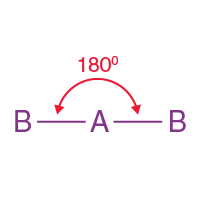Linear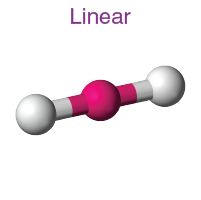BeF2, BeCl2
AB3 3 0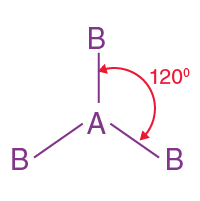Trigonal planar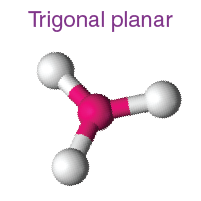BF3, BCl3
AB2L 2 1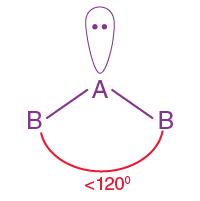Trigonal planar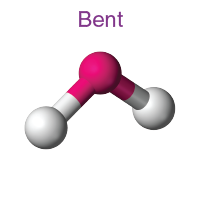Trigonal planar

SO2
AB4 4 0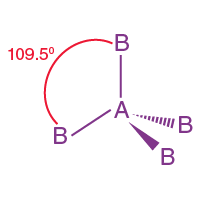Tetrahedral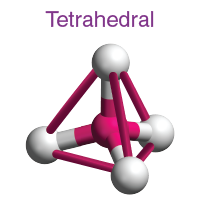CH4, CCl4
AB3L 3 1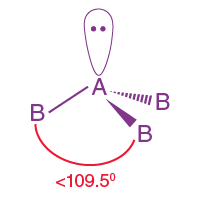Tetrahedral

Pyramidal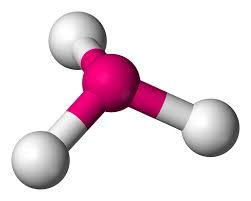NH3
AB2L2 2 2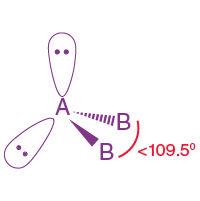TetrahedralH2O
AB5 5 0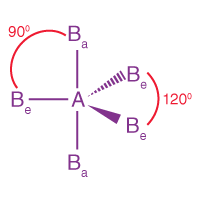Trigonal bipyramidal

l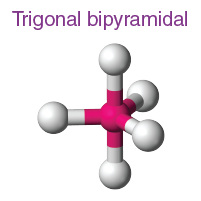PF5
AB4L 4 1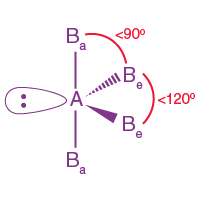Trigonal bipyramidal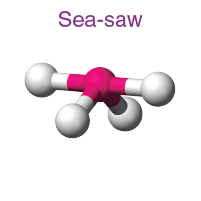SF4
AB3L2 3 2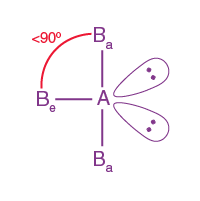Trigonal bipyramidal

T- shaped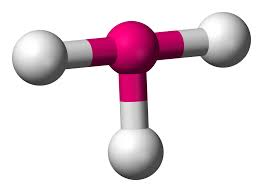ClF3
AB2L3 2 3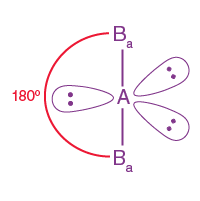Trigonal bipyramidalXeF2
AB6 6 0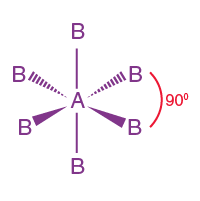Octahedral

Octahedral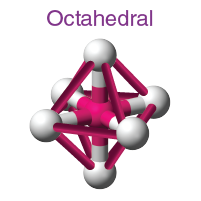SF6, TeF6
AB5L 5 1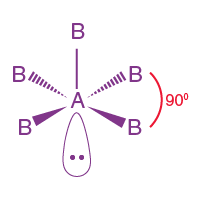Octahedral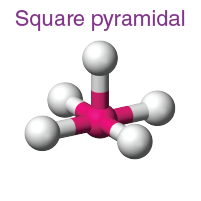ClF5
AB4L2 4 2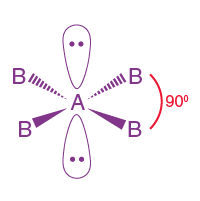Octahedral

Square planar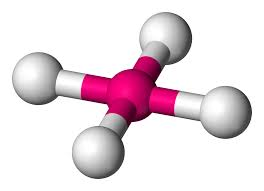XeF4

## Frequently Asked Questions on Molecular Geometry

Q1

### What are the 5 molecular geometries?

The 5 molecular geometries are linear, trigonal planar, tetrahedral, trigonal bipyramidal and octahedral.

Q2

### What is the molecular geometry of H2O?

In the H2O molecule, oxygen is the central atom. The valence electron of oxygen is six. Two of these electrons form bonds with two hydrogen atoms. Thus in H2O, the central atom contains two bond pairs and two lone pairs electrons. So the geometry is tetrahedral but the shape is bent.

Q3

### What is the molecular geometry of PH3?

The PH3 molecule has a tetrahedral geometry. The central P atom contains three bond pair electrons and one lone pair electrons.

Q4

### What is the geometry of a molecule?

Molecular geometry is the three-dimensional arrangement of the atoms that constitute a molecule. It gives information about the general shape of the molecule as well as bond lengths, bond angles, torsional angles and any other geometrical parameters that determine the position of each atom.

Q5

### What is the difference between electron geometry and molecular geometry?

Molecular geometry is described as the 3D arrangement of atoms in a molecule, normally relative to a single central atom. Whereas, electron geometry is the 3D arrangement of electron pairs around a central atom, whether bonding or non-bonding.